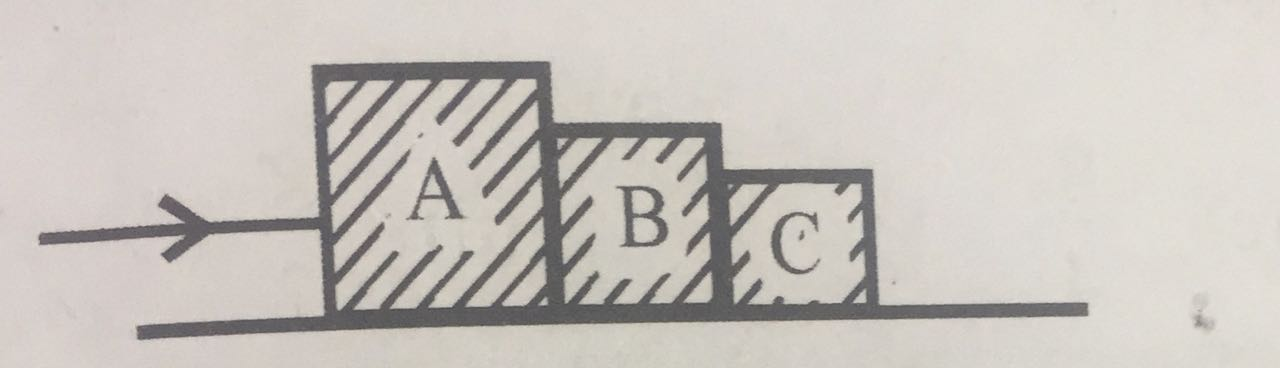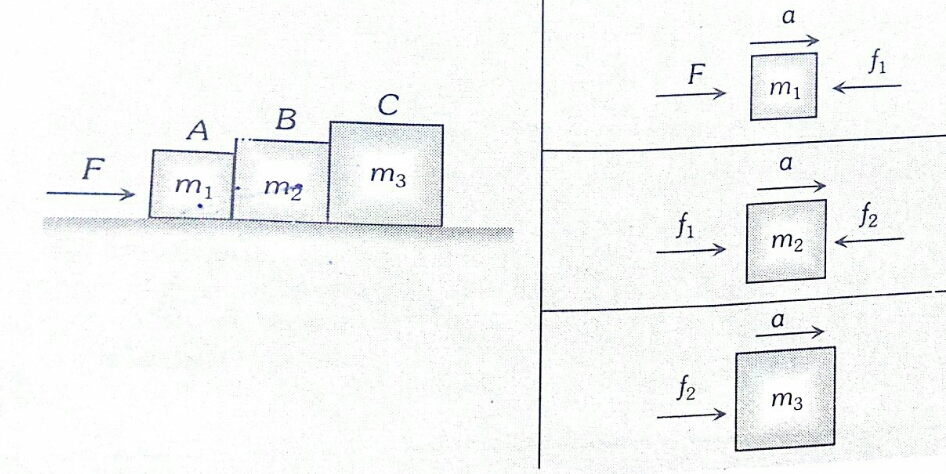#### Three blocks A, B and C, of masses 4 kg, 2 kg and 1 kg respectively, are in contact on a frictionless surface, as shown. If a force of 14 N is applied on the 4 kg block, then the contact force between A and B is:Option 1) 6 N Option 2) 8 N Option 3) 18 N Option 4) 2 NAs we learnt in

When 3 Blocks are in Contact -- whereinF = m a

a = F/m = 14 |(4+2+1) = 2m| 1s

F = ma

F = (2+1) 2

F =32 = 6 N

Contact force between A & B is used to move the bodies B & C only.

Option 1)

6 N

Correct

Option 2)

8 N

Incorrect

Option 3)

18 N

Incorrect

Option 4)

2 N

Incorrect

#### prateek